# About numbers 151 and 153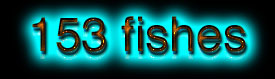First loaded on the 16th of November 2003

2010/03/31 last updating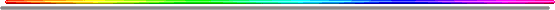http://www.bibleetnombres.online.fr/153us.htm

or"Albert Einstein" = 153

The main goal is to explain Biblical numbers with a slight "French touch" through a few fragments extracted from the main chapter dedicated to the number 153. But many remarks and paragraphs have been erased from the original pages as far as they only can be understood in French, including meaning, sound and something that could be called "logometry".

" [...] And the waters prevailed on the earth a hundred and fifty days. And God remembered Noah, and all the animals, and all the cattle that were with him in the ark; and God made a wind to pass over the earth, and the waters subsided. And the fountains of the deep and the windows of heaven were closed, and the pour of rain from heaven was stopped. And the waters retired from the earth, continually retiring; and in the course of a hundred and fifty days the waters abated. And the ark rested in the seventh month, on the seventeenth day of the month, on the mountains of Ararat. (Genesis 7/24 and 8/1-4).

If A = 1, B = 2, C = 3...
Jesus Christ " = 151

" Holy Spirit " = 151

" PATER NOSTER " = 151

Quite often through the Holy Scriptures, numbers appear to be explicative, just like watermarks, even if they stand as 90% hidden icebergs by the surrounding waters. With these Genesis' verses, it's suggested with Genesis 7/24 that a week is seven days and a day 24 hours long. The seventh hour of the seventh day is also the 151rst hour of the same week. And through these words, it's quite obvious that Our World "restarted" on a 151rst Day. " Holy Spirit " = 151 is translated from the French " Saint-Esprit " = 15O

Due to the French (UE) Summer time, the 153rd hour of the week according to a clock is at the same "time", the 151rst "sun" hour.

That's a short cut to prove how much numbers 151 and 153 are intimately linked to Our Lord Jesus-Christ and the Church Rapture. The 1st of June 2000, closing the last 20th Century and Second Millenary at the same time, was both the "feast of Ascension" and the "153rd " Day of the year...

Daniel 5/7 and 5/14-16

[144th verse] 7 The king cried aloud to bring in the astrologers, the Chaldeans, and the soothsayers. And the king spake, and said to the wise men of Babylon, Whosoever shall read this writing, and shew me the interpretation thereof, shall be clothed with scarlet, and have a chain of gold about his neck, and shall be the third ruler in the kingdom.

[151rst verse] 14 I have even heard of thee, that the spirit of the gods is in thee, and that light and understanding and excellent wisdom is found in thee.

[144th verse] 15 And now the wise men, the astrologers, have been brought in before me, that they should read this writing, and make known unto me the interpretation thereof: but they could not shew the interpretation of the thing:

[153rd verse] 16 And I have heard of thee, that thou canst make interpretations, and dissolve doubts: now if thou canst read the writing, and make known to me the interpretation thereof, thou shalt be clothed with scarlet, and have a chain of gold about thy neck, and shalt be the third ruler in the kingdom.

Such arrangements of opposed meanings are self explicative and don't need to be commented.153153...   153153 is the smallest abundant odd ending with the number 3. 153153, 351351, 513513 are all abundant odds.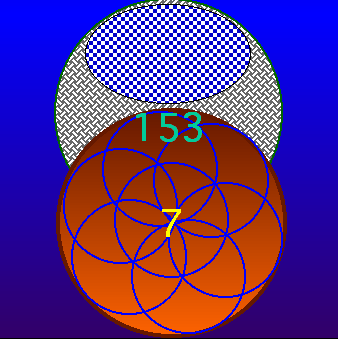153 fishes in a net caught by 7 fisher-apostles   " [...] After these things Jesus manifested himself again to the disciples at the sea of Tiberias. And he manifested himself thus. There were together Simon Peter, and Thomas called Didymus, and Nathanael who was of Cana of Galilee, and the sons of Zebedee, and two others of his disciples. Simon Peter says to them, I go to fish. They say to him, We also come with thee. They went forth, and went on board, and that night took nothing. And early morn already breaking, Jesus stood on the shore; the disciples however did not know that it was Jesus. Jesus therefore says to them, Children, have ye anything to eat? They answered him, No. And he said to them, Cast the net at the right side of the ship and ye will find. They cast therefore, and they could no longer draw it, from the multitude of fishes. That disciple therefore whom Jesus loved says to Peter, It is the Lord. Simon Peter therefore, having heard that it was the Lord, girded his overcoat on him (for he was naked), and cast himself into the sea; and the other disciples came in the small boat, for they were not far from the land, but somewhere about two hundred cubits, dragging the net of fishes. When therefore they went out on the land, they see a fire of coals there, and fish laid on it, and bread. Jesus says to them, Bring of the fishes which ye have now taken. Simon Peter went up and drew the net to the land full of great fishes, a hundred and fifty-three; and though there were so many, the net was not rent. (John 21/1-11). 153 days and 7 months constitute a whole year as far as, July, the Seventh month ends at 153 days from the end of a (overleap or not) Year. Just remember: the seventh month, on the seventeenth day of the month, on the mountains of Ararat. (Genesis 8/4)..." [...] Again, the kingdom of heaven is like unto a net, that was cast into the sea, and gathered of every kind: 48 Which, when it was full, they drew to shore, and sat down, and gathered the good into vessels, but cast the bad away. So shall it be at the end of the world: the angels shall come forth, and sever the wicked from among the just, 50 And shall cast them into the furnace of fire: there shall be wailing and gnashing of teeth... (Matthew 13/47-50)

This NET is INTERNATIONAL or an "INTERNET" to put it in other words...John the Evangelist in Patmos

" [...] Now there was leaning on Jesus' bosom one of his disciples, whom Jesus loved. (John 13/23)

" [...] Then she runneth, and cometh to Simon Peter, and to the other disciple, whom Jesus loved, and saith unto them, They have taken away the Lord out of the sepulchre, and we know not where they have laid him. (John 20/2)

" [...] Therefore that disciple whom Jesus loved saith unto Peter, It is the Lord. Now when Simon Peter heard that it was the Lord, he girt his fisher's coat unto him, (for he was naked,) and did cast himself into the sea. (John 21/7)

" [...] Then Peter, turning about, seeth the disciple whom Jesus loved following; which also leaned on his breast at supper, and said, Lord, which is he that betrayeth thee? (John 21/20)

"the disciple whom Jesus loved"...

The special Jesus' love for His disciple John is repeatedly quoted in many verses and it cannot be considered as due to a pure hazard but rather as a specific emphasis. Consequently, if Our Lord has shown this special relation with John, we must pay a closer attention to the fact that numbers 153 and 666 are specifically treated by John throughout his writings in the New Testament. It's part of the disclosure of Daniel's former sealed writings who once declared:

" [...] But thou, O Daniel, shut up the words, and seal the book, even to the time of the end: many shall run to and fro, and knowledge shall be increased. (Daniel 12/4)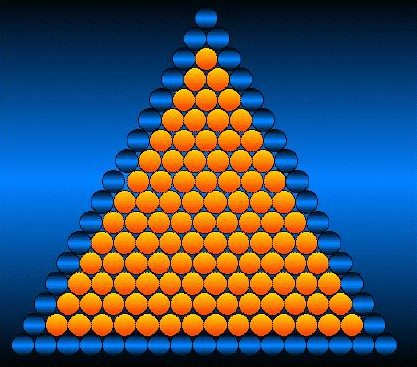As follows...:

The addition also called "Pythagorician sum" of the first 17 numbers gives 153;

1 + 2 + 3 + 4 + 5 + 6 + 7 + 8 + 9 + 10 + ... + 17 = 153

or

17 x 9 = 153

and

153 + 17 = 170

As far as 9 = 1 + 5 + 3

1 + 5 + 3 = 9 = (3)2 and the sum of all dividers of number 153 delivers a new perfect square number as far as:

1 + 3 + 9 + 17 + 51 = 81 = (9)2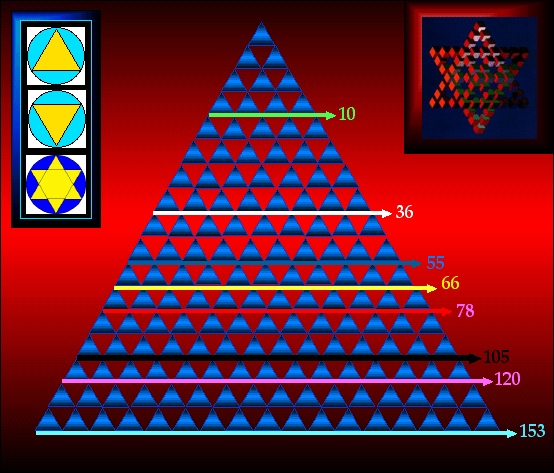The David Star is not Biblical with its occult origins. It's constituted with two equilateral triangles. This equilateral triangle is built with 153 equilateral triangle elements revealing 17 triangles on each side.

6: 1 + 2 + 3 = 1 x 2 x 3 = 6

 1 2 3 6 3 1 2 6 2 3 1 6 6 6 6

10: Pythagore's Tetractys or "TOP TEN" (10 horns) ruled by the 11th horn (Antichrist).

36: The addition of the first 36 numbers from 1 to 36 gives 666.

55: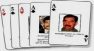11 x 5. Satan = 55. (Antichrist is the 11th horn = rebellion) See WTC 9/11 and president Bu\$h with his 55 Gulf War II cards game.

66: 66 Bible books

78: 78 Tarot cards. See president Bu\$h's Gulf War II Declaration on the 78th day of 2003.

105: 105 days = 2520 hours, 2520 being a symbolical number of the "Great Tribulation" and/or "Day of The Lord".

120; 120 years during which Noah built his ark.

Several other number 120 quotations can be located in the scriptures. Moses was 120 years old when he died (De 34/7), Hiram had sent 120 golden talents to the King (1 Kings 9/14), The Queen of Seba gave 120 to Salomon (1 Kings 10/10), There were 120 trumpet sounding sacrificators (2 Ch 5/12)...

" [...] And she gave the king an hundred and twenty talents of gold, and of spices very great store, and precious stones: there came no more such abundance of spices as these which the queen of Sheba gave to king Solomon. (1 Kings 10/10).

" [...] Also at the same time Solomon kept the feast seven days, and all Israel with him, a very great congregation, from the entering in of Hamath unto the river of Egypt. (2 Chron. 7/8)

Those indications are quite precious if we consider that the queen of Sheba gave an hundred and twenty talents of gold to king Solomon, which used to get six hundred and threescore and six talents of gold per year.

" [...] Now the weight of gold that came to Solomon in one year was six hundred threescore and six talents of gold, (1 Ki 10/14)
" [...] Now the weight of gold that came to Solomon in one year was six hundred and threescore and six talents of gold; (2 Ch 9/13)

Important remark:

" [...] for it is the number of a man; and his number is Six hundred threescore and six. (Revelation. 13/18)

The word "number" is repeated twice in this section.

" [...] Car c'est un nombre d'homme, et son nombre est six cent soixante-six. (Revelation 13/18).

These letters, when translated into French, give a total amount of exactly 666.

" [...] Let us be glad and rejoice, and give honour to him: for the marriage of the Lamb is come, and his wife hath made herself ready. And to her was granted that she should be arrayed in fine linen, clean and white: for the fine linen is the righteousness of saints. And he saith unto me, Write, Blessed are they which are called unto the marriage supper of the Lamb. And he saith unto me, These are the true sayings of God. (Revelation. 19/7-8).

These feast seven days and six hundred and threescore and six talents of gold are quite representative of the marriage of the Lamb during the seven years long week Tribulation.

" [...] And I saw as it were a sea of glass mingled with fire: and them that had gotten the victory over the beast, and over his image, and over his mark, and over the number of his name, stand on the sea of glass, having the harps of God. (Revelation 15/2)

This verse 15/2, between 15/1 and 15/3 is quite capital as far as that there are only 153 verses left up to the end of the Bible.

" [...] And they sing the song of Moses the servant of God, and the song of the Lamb, saying, Great and marvellous are thy works, Lord God Almighty; just and true are thy ways, thou King of saints. (Revelation 15/3)

That simply means that this verse 15/3 is also verse [-153]!

4 Who shall not fear thee, O Lord, and glorify thy name? for thou only art holy: for all nations shall come and worship before thee; for thy judgments are made manifest.
5 And after that I looked, and, behold, the temple of the tabernacle of the testimony in heaven was opened:

and verse 15/5 is also verse [-151]!

----------------

Resulting from a mirroring addition, 153 + 351 = 504 and (504)2 = 288 x 882, we get these two mirror numbers.

153 and 351 are respectively the sum of the 17 and 26 first numbers. Our alphabet is composed of 26 letters and this square number (504)2 stands as the smallest which enable to get two inverted numbers.

153 is also divisible by its own components sequence just as follows:

153 / [1+5+3] = 17 and, as it can be checked on the Pascal triangles picture, one of the inverted sequence components divided by the remaining two other components, gives 153 = 3 x 51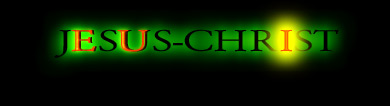roll over picture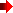The simple addition of the vowels extracted from the name of Jesus-Christ gives e-u = 26 and i = 9The 26th of the 9th month is also the 269th day of a (non overleap) year.26 letters and 9 figures enable anyone to make a copy of a whole Bible.

Permutations of 153 allow 6 different possible permutations, with equivalencies such as:

153 + 315 + 531 = 999 = 351 + 135 + 513

And naturally: 153 + 315 + 531 + 351 + 135 + 513 = 1998 = 666 + 666 + 666

We'll come back on that subject...

Table of the 50 first triangular numbers

 1 = 1 2 = 3 3 = 6 4 = 10 5 = 15 6 = 21 7 = 28 8 = 36 9 = 45 10 = 55 11 = 66 12 = 78 13 = 91 14 = 105 15 = 120 16 = 136 17 = 153 18 = 171 19 = 190 20 = 210 21 = 231 22 = 253 23 = 276 24 = 300 25 = 325 26 = 351 27 = 378 28 = 406 29 = 435 30 = 465 31 = 496 32 = 528 33 = 561 34 = 595 35 = 630 36 = 666 37 = 703 38 = 741 39 = 780 40 = 820 41 = 861 42 = 903 43 = 946 44 = 990 45 = 1035 46 = 1081 47 = 1128 48 = 1176 49 = 1225 50 = 1275

- One can notice that if 17 is a triangular root number of 153, 26 is also a triangular root number of the reversed number 351.

 = 4 + 4

3 = 4913 and 4+9+1+3 = 17

and coming back to 170;

170 : 1110 = 0, 153 153 153... = 102 : 666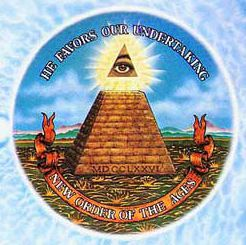Pyramid on the American 1\$ dollar Bill

This number 1110 is underlined on the occult (MDCCLXXVI) 1776 one dollar Bill Great Seal as far as 1110 + 666 = 1776 (see tables and pictures which speak for themselves at US great Seal part I, II and III French pages) and converting 1110 from a sexagesimal into a centesimal system, in other words from a former Babylonian to a modern Occidental one, we get 1110 x 0.60 = 666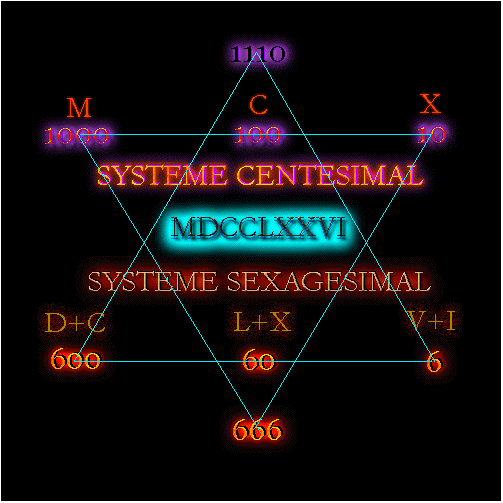— The U.S. Great Seal has been created on the 1st of May 1776, a [1 - 5 - 3] combination because 1+7+7+6 = 21 and 2+1 = 3 according to the French dating system — The occult roots are easily traceable through simple equivalencies such as:

666 : 2 = 222 and 1998 = 666 x 3

153 + 153 + 360 + 666 + 666 = 1998 = 666 + 666 + 666

153 + 315 + 531 + 351 + 135 + 513 = 1998 = 666 + 666 + 666

1776 + 153 = 1929 or a planned Economical Crisis.

Daniel's week is 2595 days long.

" [...] Blessed is he that waiteth, and cometh to the thousand three hundred and thirty-five days! (Daniel 12/12)

1260 days + 1335 days = 2595 days

2595 - 666 = 192911th of September...

A simple date reveals more about many eschatological "events" such as the WTC lie and the true meaning of the green enslaving chain-key marked "dolor" bank note, known as the "dollar".

360°, the measure of a circle, after a simple permutation, 360 ==>> 306, allows to write:

360 + 306 = 666 = 153 + 360 + 153

or

666 = 153 + 153 + 54 + 153 + 153

and to make it simpler, another permutation gives;

666 = 153 + 513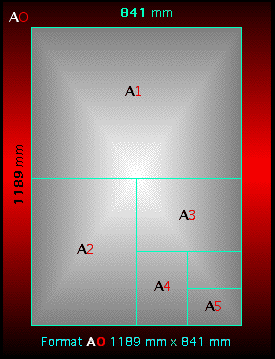The whole Bible OT + NT being composed of 1189 chapters, it's interesting to note that the standardised ISO AO paper Format with a surface of 1 square meter is normalised to a 1189 mm x 841 mm surface.

According to Daniel's and John's calculations in their writings, the Tribulation Week of (360 days) 7 Years is composed of 1260 days x 2 = 2520 (+ a special transitory period of 75 days for Daniel as seen before). Our French 21 x 29.7 A4 paper format, after conversion into 210 mm x 297 mm reveals these eschatological measures:Six vertically set A4 paper sheets reach 210 mm x 6 = 1260 mmTwo horizontally set A4 paper sheets occupy 297 mm x 2 = 594 mm in space and 594 + 666 = 1260Three vertically set A3 paper sheets reach 420 mm x 3 = 1260 mmTwo horizontally set A3 paper sheets occupy 297 mm x 2 = 594 mm in space and 594 + 666 = 1260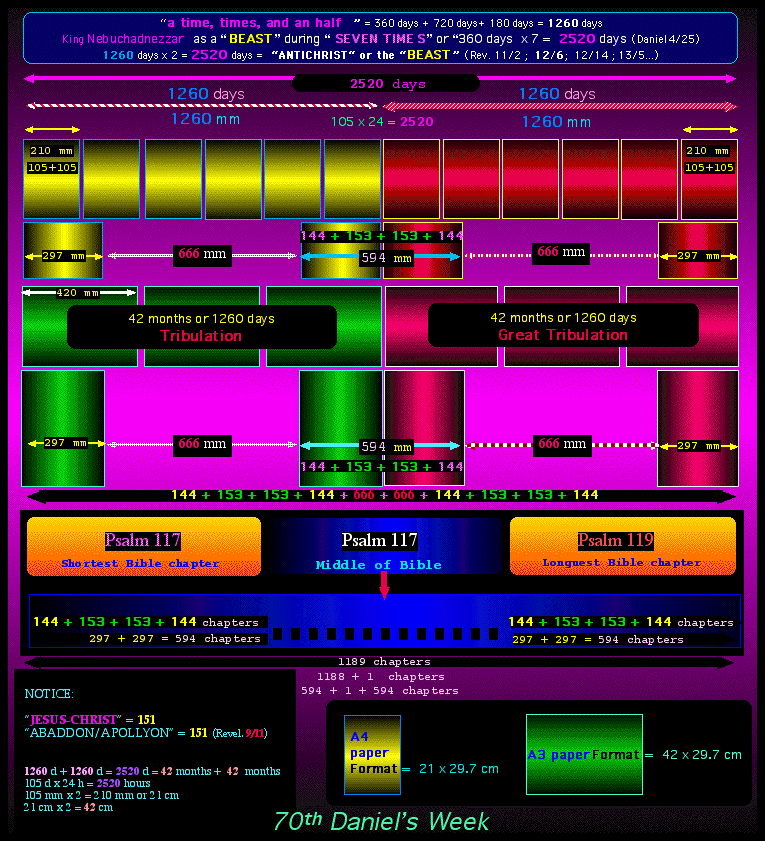For a black & white Gif picture, please click on this link:

We notice that:

297 = 144 + 153

2 = [88 209] and [88 + 209] = 297

" [...] And he measured the wall thereof, an hundred and forty and four cubits, according to the measure of a man, that is, of the angel. (Revelation 21/17)

This number has many distinctive features:

First example:

(1)3 + (5)3 + (3)3 = 1 + 125 + 27 = 153.

Only three other numbers have this distinctive feature :

370 = (3)3 + (7)3 + (0)3 = 27 + 343 + 0

371 = (3)3 + (7)3 + (1)3 = 27 + 343 + 1

407 = (4)3 + (0)3 + (7)3 = 64 + 0 + 343

Permutations will give:

 370 + 307 + 703 + 730 + 037 + 073 = 2220 2220/6 = 370 371 + 317 + 137 + 173 + 713 + 731 = 2442 2442/6 = 407 407 + 470 + 047 + 074 + 704 + 470 = 2442 2442/6 = 407

Jésus = 74

 74 =  3 +  3 343 + 64 = 407 407 =  3 +  3 +  3 64 + 0 + 343 = 407 407 =  3 +  3 +  3 64 + 0 + 343 = 407...

Christ = 77

 77 =  3 +  3 343 + 343 = 686 686 =  3 +  3 +  3 216 + 512 + 216 = 944 944 =  3 +  3 +  3 729 + 64 + 64 = 857 857 =  3 +  3 +  3 512 + 125 + 343 = 980 980 =  3 +  3 +  3 729 + 512 + 0 = 1241 1241 =  3 +  3 +  3 +  3 1 + 8 + 64 + 1 = 74 74 =  3 +  3 343 + 64 = 407 407 =  3 +  3 +  3 64 + 0 + 343 = 407...

We know that 74 days give an amount of 1776 hours.

With the Greek values of the name of JESUS-CHRIST, we find:

JESUS = 888 and CHRIST = 1480

 888 =  3 +  3 +  3 512 + 512 + 512 = 1536 1536 =  3 +  3 +  3 +  3 1 + 125 + 27 + 216 = 369 369 =  3 +  3 +  3 27 + 216 + 729 = 972 972 =  3 +  3 +  3 729 + 343 + 8 = 1080 1080 =  3 +  3 +  3 +  3 1 + 0 + 512 + 0 = 513 513 =  3 +  3 +  3 125 + 1 + 27 = 153 153 =  3 +  3 +  3 1 + 125 + 27 = 153...

 1480 =  3 +  3 +  3 +  3 1 + 64 + 512 + 0 = 577 577 =  3 +  3 +  3 125 + 343 + 343 = 811 811 =  3 +  3 +  3 512 + 1 + 1 = 514 514 =  3 +  3 +  3 125 + 1 + 64 = 190 190 =  3 +  3 +  3 1 + 729 + 0 = 730 730 =  3 +  3 +  3 343 + 27 + 0 = 370 370 =  3 +  3 +  3 27 + 343 + 0 = 370...

370 x 24 h = 8880 hours. This amount of time calculated in hours is not a coïncidence as far as 8880 hours represent the 370 days spent by Noah in his ark.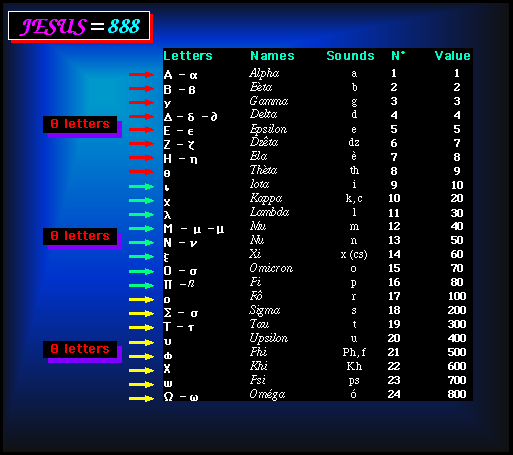Note that the name of Jesus is revealed through the Greek Alphabet...

JESUS-CHRIST = 888 + 1480 = 2368

 2368=  3 +  3 +  3 +  3 8 + 27 + 216 + 512 = 763 763 =  3 +  3 +  3 343 + 216 + 27 = 586 586 =  3 +  3 +  3 125 + 512 + 216 = 853 853 =  3 +  3 +  3 512 + 125 + 27 = 664 664 =  3 +  3 +  3 216 + 216 + 64 = 496 496 =  3 +  3 +  3 64 + 27 + 216 = 307 307 =  3 +  3 +  3 27 + 0 + 343 = 370... 370 =  3 +  3 +  3 27 + 343 + 0 = 370...

The second temple has been destroyed by Titus in 70 A.D.

70 = (7)3 + (0)3 = 343 + 0

(3)3 + (4)3 + (3)3 = 118

(1)3 + (1)3 + (8)3 = 514

(5)3 + (1)3 + (4)3 = 190

(1)3 + (9)3 + (0)3 = 730

(7)3 + (3)3 + (0)3 = 370The sum of all dividers of the number 70, including 70 itself, amounts to 144 as follows:

1 + 2 + 5 + 7 + 10 + 14 + 35 + 70 = 144

Another example with 2003 reveals the terminal number 371:

(2)3 + (0)3 + (0)3 + (3)3 = 8 + 0 + 0 + 27 = 35
(3)3 + (5)3 = 27 + 125 = 152
(1)3 + (5)3 + (2)3 = 1 + 125 + 8 = 134
(1)3 + (3)3 + (4)3 = 1 + 27 + 64 = 92
(9)3 + (2)3 = 729 + 8 = 737
(7)3 + (3)3 + (7)3 = 343 + 27 + 343 = 713
(7)3 + (1)3 + (3)3 = 343 + 1 + 27 = 371 et (3)3 + (7)3 + (1)3 = 371

with increasing value of [(.)0 + (.)1 + (.)2] exponents, we can write:

(1)0 + (5)1 + (3)2 = 1 x 5 x 3

and

(1)0 + (5)1 + (3)2 = 15

(1)1 + (5)2 + (3)3 = 53

" [...] And the city lieth foursquare, and the length is as large as the breadth: and he measured the city with the reed, twelve thousand furlongs. The length and the breadth and the height of it are equal. And he measured the wall thereof, an hundred and forty and four cubits, according to the measure of a man, that is, of the angel. (Revelation 21/16-17)

The cube exponent is related to the New Celestial Jerusalem which is certainly shaped as a cube (and not as a dome or a pyramid, whose shapes are not present in the first and second temples).

" [...] And this is the fashion which thou shalt make it of: The length of the ark shall be three hundred cubits, the breadth of it fifty cubits, and the height of it thirty cubits. A window shalt thou make to the ark, and in a cubit shalt thou finish it above; and the door of the ark shalt thou set in the side thereof; with lower, second, and third stories shalt thou make it. (Ge 6/15-16)

This word "cubits" is first quoted in the Genesis' 153rd verse and the two last "cubits" occurrences are quoted in John's Gospel and his book of Revelation as follows:

" [...] And the other disciples came in a little ship; (for they were not far from land, but as it were two hundred cubits,) dragging the net with fishes. (John 21/8)
" [...] And he measured the wall thereof, an hundred and forty and four cubits, according to the measure of a man, that is, of the angel. (Revelation 21/17)

A Catholic Rosary (chaplet) is composed of 153 (non Biblical and highly parodying) beads and has been, through the crusades and religion wars, adapted from the 99 beads Muslim chaplet, the Muslim one being copied on the Tibetan 108 beads (skull pieces) chaplet.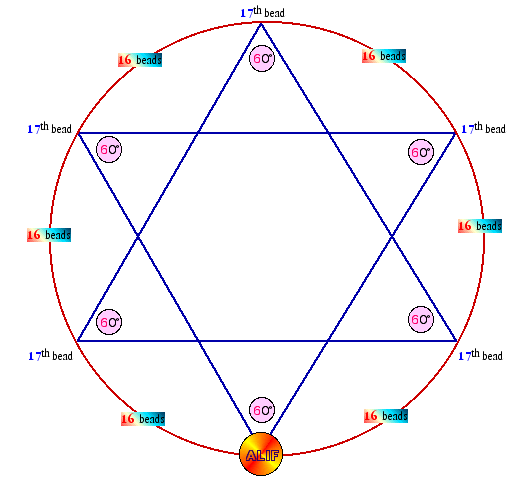Laying the Muslim chaplet with its 99 "operative" beads into a circle shape, we get six points and the composition of a David star. Number 666 stands behind 60° angle measures and 16 beads sequences. (That's just a way to explain why men are claiming to fight in the Name of God but under the banner of Satan in reality. The mysterious and enigmatic crop circles are full of such subliminal infamous suggestions). This figure shows the process of (continuous) counting of "prayers" with 99 beads, (16 beads + 17th bead) 33 by 33 sequenced and separated by three "blank" beads including the symbolical ALIF in such a way that the loop has no beginning or/and no end.

153 x 99 = 15147

This 151/153 connection is revealed through such quite simple calculations.

151 + 153 = 304 days

This amount of 304 days, (non overleap years), reveals the "Feast" of Halloween during which children are "traditionally" ( but unwillingly at first) entangled and involved. Witchcraft is the most suitable word to qualify this "feast".

151/153

roll over picture

The ASCII equivalencies table for the following numbers are;

[1 - 5 - 1] equivalent to [49 + 53 + 49] = 151

[1 - 5 - 3] = [49 + 53 + 51] = 153

[1 - 0 - 8] = [49 + 48 + 56] = 153

[1 - 3 - 5] = [49 + 51 + 53] = 153

[1 - 4 - 4] = [49 + 52 + 42] = 153

[2 - 1 - 6] = [50 + 49 + 54] = 153French book front cover : "Le martin pêcheur" by Marie Claude & Jean-Gilles Baillet   Two words extracted from the French Dictionary "oiseau" ("bird") and "Christ" are absolutely unique as far as they are respectively composed of 5 vowels and one single consonant or 5 consonants and one single vowel. A bird name like "martin-pêcheur" ("King Fisher") corresponds to an inserted 151 numerical sequence "75 + 76". This bird is traditionally attached to John the Apostle. Jesus-Christ ("74 + 77") is both a King and a Fisher.

Coming back to numbers 153 and 1776 which are connected to the US Dollar, we can add as follows:

(1)3 + (7)3 + (7)3 + (6)3 = 1 + 343 + 343 + 216 = 903

(9)3 + (0)3 + (3)3 = 729 + 0 + 27 = 756

(7)3 + (5)3 + (6)3 = 343 + 125 + 216 = 684

(6)3 + (8)3 + (4)3 = 216 + 512 + 64 = 792

(7)3 + (9)3 + (2)3 = 343 + 729 + 8 = 1080

(1)3 + (0)3 + (8)3 + 0)3 = 1 + 0 + 512 + 0 = 513

(5)3 + (1)3 + (3)3 = 153

(1)3 + (5)3 + (3)3 = 153

The Greek alphanumeric value of JESUS = 888

 888 =  3 +  3 +  3 512 + 512 + 512 = 1536 1536 =  3 +  3 +  3 +  3 1 + 125 + 27 + 216 = 369 369 =  3 +  3 +  3 27 + 216 + 729 = 972 972 =  3 +  3 +  3 729 + 343 + 8 = 1080 1080 =  3 +  3 +  3 +  3 1 + 0 + 512 + 0 = 513 513 =  3 +  3 +  3 125 + 1 + 27 = 153

All numbers which can be divided by 3 reach this result of this 153 specific process according to the same calculation method.

And as it could be expected, 666 does not escape to this "miraculous fishing" sign:

 666 =  3 +  3 +  3 216 + 216 + 216 = 648 648 =  3 +  3 +  3 216 + 64 + 512 = 792 792 =  3 +  3 +  3 343 + 729 + 8 = 1080 1080 =  3 +  3 +  3 +  3 1 + 0 + 512 + 0 = 513 513 =  3 +  3 +  3 125 + 1 + 27 = 153

Another particularity of this process reveals that any number lower than 2000 can generate a greater number during the calculating process progression. As a simple example, 99 will give:

 99 =  3 +  3 729 + 729 = 1458 1458 =  3 +  3 +  3 +  3 1 + 64 + 125 + 512 = 702 702 =  3 +  3 +  3 343 + 0 + 8 = 351 351 =  3 +  3 +  3 27 + 125 + 1 = 153

Through this example, the intermediary 1458 is greater than the starting 99.

If we try to apply the same process to any number greater than 2000, we discover that it never happens anymore:

 2001 =  3 +  3 +  3 +  3 8 + 0 + 0 + 1 = 9

Or:

 3009 =  3 +  3 +  3 +  3 27 + 0 + 0 + 729 = 756 756 =  3 +  3 +  3 343 + 125 + 216 = 684 684 =  3 +  3 +  3 216 + 512 + 64 = 792 792 =  3 +  3 +  3 343 + 729 + 8 = 1080 1080 =  3 +  3 +  3 +  3 1 + 0 + 512 + 0 = 513 513 =  3 +  3 +  3 125 + 1 + 27 = 153

From and over 2000, the treated intermediary numbers are always lower than the starting number. From 1 to 2000 there are exactly 666 numbers which match with that kind of operation!

Numbers added from 1 to 2000 = 2001.000, equivalent to 2001 x 1000 in another way.

With this particularity in mind, our Millenium has consequently a special "added" eschatological feature."M" is the 13rd letter of the alphabet and 13 is the number of rebellion and if we apply the same process:

 13 =  3 +  3 1 + 27 = 28 28 =  3 +  3 8 + 512 = 520 520 =  3 +  3 +  3 125 + 8 + 0 = 133 133 =  3 +  3 +  3 1 + 27 + 27 = 55 55 =  3 +  3 125 + 125 = 250 250 =  3 +  3 +  3 8 + 125 + 0 = 133 133 =  3 +  3 +  3 1 + 27 + 27 = 55 55 =  3 +  3 125 + 125 = 250 250 =  3 +  3 +  3 8 + 125 + 0 = 133…

infernal circle!

It never ends...

1998 = 666 x 3 and considering the 360 degrees of a circle, we get as an equivalence for 1998:

153 + 153 + 360 + 666 + 666

The 6 (demoniac) so called "Our Lady" apparitions of Fatima started on the 13th of May 1917, 133rd day of that year. They occurred on each 13th day of June, July, August, September and finally October and lasted 153 days. Just parody, always parody!

Numbers, we have already seen, from 1 to 17 give 153, and the integrated square prime numbers amount to 666 because:

2 + 2 + 2 + 2 + 2 + 2 + 2

4 + 9 + 25 + 49 + 121 + 169 + 289 = 666Click to enlarge

Many Biblical expressions, in their original Greek alphanumeric value state associated to "fishes" and "fishing" can be divided by 153:

Greek Words and expressions are multiple of 153 like: " fishes ", " the net  ", = 1224 = 153 x 8, " multitude of fishes " = 153 x 16, " fisher of men " = 153 x 14, " casting the net into the sea " = 153 x 20, etc.

The addition of the 17 first numbers amount to 153 and such a process being applied to our alphabet, we get 351 as a value for the 26th and last letter.

 A = 1 B = 3 C = 6 D = 10 E = 15 F = 21 G = 28 H = 36 I = 45 J = 55 K = 66 L = 78 M = 91 N = 105 O = 120 P = 136 Q = 153 R = 171 S = 190 T = 210 U = 231 V = 253 W = 276 X = 300 Y = 325 Z = 351

The Exponential (n !) values of the 5 first figures amount also to 153 :

 Figures n ! = total 1 1 = 1 2 2 = 3 3 6 = 9 4 24 = 33 5 120 = 153

The calculation process is as follows:

1! = 1 x 1 = 1

2! = 1 x 2 = 2

3! = 1 x 2 x 3 = 6

4! = 1 x 2 x 3 x 4 = 24

5! = 1 x 2 x 3 x 4 x 5 = 120

120 has already been treated with the 153 Pascal's triangle.

If, as a custom by Satanists, we reverse the alphabetical values of our 26 letters, we get "ANTECHRIST" = 153 according to the following table:

 A = 26 B = 25 C = 24 D = 23 E = 22 F = 21 G = 20 H = 19 I = 18 J = 17 K = 16 L = 15 M = 14 N = 13 O = 12 P = 11 Q = 10 R = 9 S = 8 T = 7 U = 6 V = 5 W = 4 X = 3 Y = 2 Z = 1

Other operations: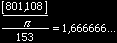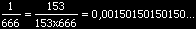According to the French date system, every 9th year or 108th month, the 1st, 10th, 19th and 28th of May correspond to [1-5-1] or [1-5-3] sequences... Such sequences, in the Anglo-Saxon system, would correspond to the 5th, 14th and 31st of January and October.

These sequences [1-5-3] have been available in 1947, 1956, 1965, 1974, 1983, 1992... 2001, and in the future 2010, 2019...

In 1956, an overleap year with 366 days, the 1st of May (+ 10th, 19th and 28th) built a sequence [1-5-3] and the 1st of June was the 153rd day of that year.According to an acceptable and probable tradition, it is said that Jesus' ministry lasted 153 weeks or 153 Sabbaths.

This number 153 is certainly linked to the symbolical total amount of "raptured" people and/or saved people since the beginning of humanity up to the Judgment and having access to new heavens and a new earth.

Extracted from the Revelation, the 153rd verse reveal a 150 to 153 days long period according to our Judeo-Christian calendar.

" [...] And to them it was given that they should not kill them, but that they should be tormented five months: and their torment was as the torment of a scorpion, when he striketh a man. And they had tails like unto scorpions, and there were stings in their tails: and their power was to hurt men five months. (Revelation 9/9-10)" [...] And after those days his wife Elisabeth conceived, and hid herself five months, saying, (Luke 1/24)

We are not really surprised to find this 150 to 153 days long period in another verse with John the baptist's mother hiding herself five months:

 Non overleap year Overleap year January 31 31 January 31 31 February 28 28 28 February 29 29 29 March 31 31 31 31 March 31 31 31 31 April 30 30 30 30 30 April 30 30 30 30 30 May 31 31 31 31 31 31 May 31 31 31 31 31 31 June 30 151 30 30 30 30 30 June 30 152 30 30 30 30 30 July 31 150 31 31 31 31 31 July 31 151 31 31 31 31 31 August 31 153 31 31 31 31 31 August 31 153 31 31 31 31 31 September 30 153 30 30 30 30 September 30 153 30 30 30 30 October 31 153 31 31 31 October 31 153 31 31 31 November 30 153 30 30 November 30 153 30 30 December 31 153 31 December 31 153 31 153 153

And, related to these "150/153" minimal and maximal values, just remember:

" [...] And the waters prevailed on the earth a hundred and fifty days. And God remembered Noah, and all the animals, and all the cattle that were with him in the ark; and God made a wind to pass over the earth, and the waters subsided. And the fountains of the deep and the windows of heaven were closed, and the pour of rain from heaven was stopped. And the waters retired from the earth, continually retiring; and in the course of a hundred and fifty days the waters abated. And the ark rested in the seventh month, on the seventeenth day of the month, on the mountains of Ararat. (Genesis 7/24 and 8/1-4).

The Torah is composed of the 5 first Bible Books (Genesis + Exodus + Leviticus + Numbers + Deuteronomy) with a total amount of 50 + 40 + 27 + 36 = 153 chapters for the first four (Genesis + Exodus + Leviticus + Numbers). The first Book is composed of 1533 verses and the fourth Book, the Book of Numbers... is composed of 36 chapters and 1+2+3+4...35+36 = 666!

153 x 12 = 1836 and 108 x 17 = 1836

1836 is linked to the following equivalence 18 x 2 = 36

In relation with numbers 108 and 144 we can develop a few particularities:

1836 x 2 = 3672 and 36 + 72 = 108

1 + 8 + 3 + 6 = 18 or [6 + 6 + 6] and 1 x 8 x 3 x 6 = 144

151 is a prime number and "prime number" translated into French "nombre premier" alphanumerically adds to... 151.

If we write 151 ("one hundred and fifty one" = "cent cinquante et un") in French, we get the following result

 c e n t c i n q u a n t e e t u n 3 5 14 20 3 9 14 17 21 1 14 20 5 5 20 21 14

The first 14 letters amount to 151 and the total of the 17 letters give 206.

The human skeleton is composed of 206 bones

" [...] And he bearing his cross went forth into a place called the place of a skull, which is called in the Hebrew Golgotha: (John 19/17) and (Mt 27/33) + (Lu 23/33)
 c e n t c i n q u a n t e t r o i s 3 5 14 20 3 9 14 17 21 1 14 20 5 20 18 15 9 19

The same calculation about number 153 ("one hundred and fifty three" = "cent cinquante trois") gives 227.

Considering the French dating system, we can analyze the following revealing sequences:

15th of March = 15/3

From this date 15/3, if we add 153 days, we get to the 15th of August, which is the (totally parodying and not Biblical) Roman Catholic feast of Assumption, a false Mary's "rapture into Heaven" never quoted in the Scriptures but related to the Babylonian Goddess known as the "Queen of Heaven". As for overleap years, this sequence is no more correct with 228 instead of 227 days.

"The Assumption" amounts to 153 in it's French translation "l'Assomption"... .

The 36 hours long blackout which first struck New York City during the night of that 227th day (with London 14 days later for a few hours at day break) and Rome on the 28th of September during the first Italian "white night" celebration, has been willingly set by the New World Order mongers as a world Masonic harbinger sign.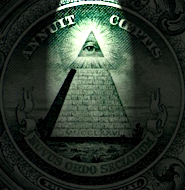We have seen that the 1st of May 1776, was a [1 - 5 - 3] combination because 1+7+7+6 = 21 and 2+1 = 3 according to the French dating system.

1776 + 227 = 2003

227 years later, the 1st of May 2003, could be written 01/05/03 according to the French dating system.

The 1st of August 1850 was the +213rd [-153rd] day of that year and could be written 1.8.50

1850 + 153 = 2003

As a war monger or a peace bringer, Hitler was a Great Liar and the same process works actually with the same proficiency at the White House.

The year 2003 is incredibly full of events connected to the number 153.

01/05/03: official end of war in Iraq according to Mr Bu\$h's own words.

02/06/03: 50 years of Queen Elisabeth II's reign, crowned in 1953 on a 153rd day).

15/08/03: 15/3 + 153 days= total NYC blackout during the whole day!...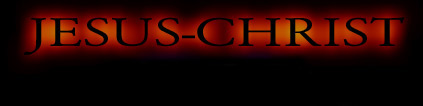roll over picture

"O" and "R", are the l5th and 18th letters in our alphabet.roll over picture

"GOLD" is translated by "OR", "ANGEL" by "ANGE" and "ORANGE" by "ORANGE" in French. "ORANGE" has consequently several meanings in French.

"JESUS-CHRIST" = 74 + 77 = 151 and the 74th - 77th days of a 365 days year are the l5th - 18th of March.

They become the 75th - 78th days of a 366 days long year and 75 + 78 = 153."Blaise Pascal" "Pascaline" or "Pascal's computer" click to enlarge

The first computing machine or "computer" was a wooden masterpiece made in the 17th Century by its creator, Blaise Pascal, both a French genius and a Christian. With this computing machine, "La Pascaline", Blaise Pascal popularized at the same time its operating system based on the "0/1" binary code which is still in use on our modern computers.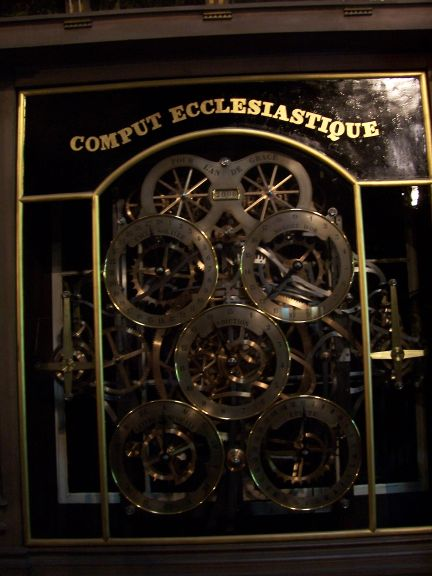ECCLESIASTICAL COMPUT

Clockwork detail

Cathédral of Strasbourg (France)

Picture credit: Alain E...

The word "computer" comes from an old French Middle Age verb "Computer" ("to count" in English) which gave finally "compter" in modern French. A "computer" is called "ordinateur" in French, deriving with this religious connotation from the verb "ordiner" which means "to organize" or "to classify according to the rules of God" in French. And "ordiner un prêtre" means "to ordain a priest". From an eschatological point of view, Internet as both a Network and an International Net is strongly attached to the French language. A few days before President G. W. Bush's waging war and exporting chaos into Iraq in March 2003, many American barking blinded journalists spread all around trash articles about France and the French "coward" position. It seems that facts give reason to us and that a doomed Bush's generation is once more fataly trapped by another one! Babylon's identity has changed!

" [...] Then said all the trees to the thorn-bush, Come thou, reign over us. And the thorn-bush said to the trees, If in truth ye anoint me king over you, come, put confidence in my shadow; but if not, fire shall come out of the thorn-bush and devour the cedars of Lebanon. (Jude 14-15)

The "thorn-bush" is the right figure to be applied to the end-time Antichrist and a recapitulation of a few facts are self-explanative about this subject.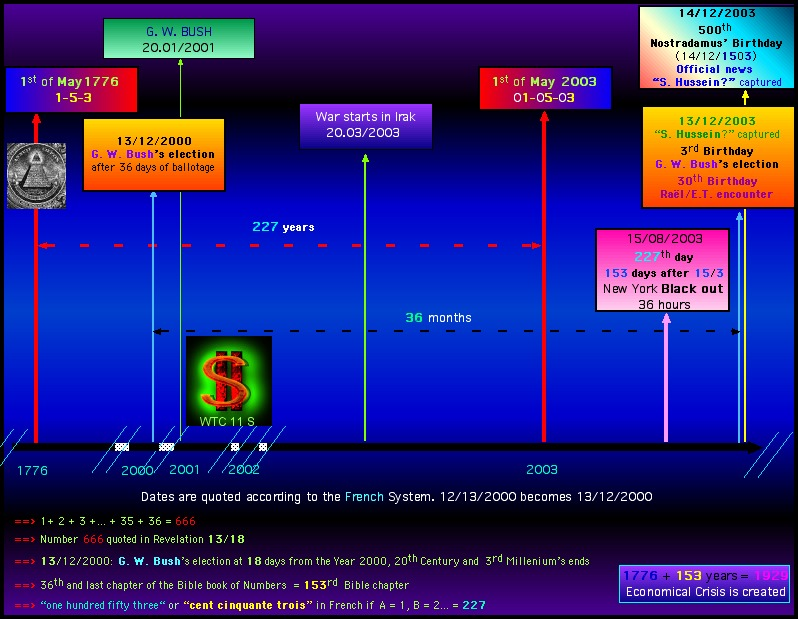The 2000 November/December US presidential elections fake lasted 36 days and 1+2+3...+35+36 = 666

" [...] for it is the number of a man; and his number is Six hundred threescore and six. (Revelation 13/18)

This verse, when translated into French as seen before, gives a total amount of 666.G. W. Bush was finally "elected" on the 13th of December 2000, 18 days before the end of a year, of a century and even a Millenary!The capture of Saddam Hussein on the 13th of December 2003, 36 months later, day for day, and its official announcement on the following day is just one trick more.This 14th of December 2003 was the 500th anniversary of Nostradamus' Birthday (14th of December 1503)...227 days after the 1rst of May 2003 (01/05/03).

" [...] The children of Adonikam, six hundred and sixty-six. (Ezr 2/13)

" [...] The children of Adonikam, six hundred and sixty-seven. (Ne 7/18)This 14th of December 2003 was also the last day of the 667th month of Israel State's existence (14th of May 1948).Is it necessary to add that the 36 hours long blackout struck New York on the 15th of August, 227th day of the year, 153 days after the 15th of March (15/3)!

President G. W. Bush's "enthronization" took place on the 20th of January 2001 and Gulf War II started on the 20th of March 2003 in Iraq.If these dates 01/20/2001 and 03/200/2003 seem to be without apparent signification, they appear to be quite more explicative in French:

20.01/2001 and 20.03/2003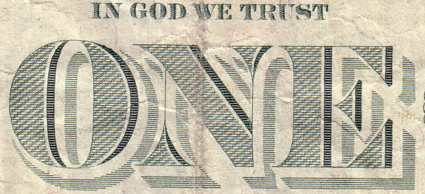"In God we trust"

"ONE"

Considering the "9/11" Event...

As far as two black shades outline the letter "O", 3 outline the letter "N" and 4 grey shades outline the letter "E", we get a total of 9 according to the sequence 2+3+4
The second layer of shades delivers the following results:

Two grey shades outline the letter "O", 5 the letter "N" and 4 the letter "E", with a result of 11 corresponding to the sequence 2+5+4...

The 9/11/2001 corresponded to the 254th day of that year...9:11' A.M. 21:11' P.M.

9/11 or 21/11?

We remember that:

370 = (3)3 + (7)3 + (0)3 = 27 + 343 + 0

371 = (3)3 + (7)3 + (1)3 = 27 + 343 + 1

407 = (4)3 + (0)3 + (7)3 = 64 + 0 + 343

879 = (8)3 + (7)3 + (5)3 = 512 + 343 + 729 = 1584

1584 = (1)3 + (5)3 + (8)3 + (4)3 = 1 + 125 + 512 + 64 = 702

702 = (7)3 + (0)3 + (2)3 = 343 + 0 + 8 = 351

351 = (3)3 + (5)3 + (1)3 = 27 + 125 + 1 = 153

459 = (4)3 + (5)3 + (9)3 = 64 + 125 + 729 = 918

918 = (9)3 + (1)3 + (8)3 = 729 + 1 + 512 = 1242

1242 = (1)3 + (2)3 + (4)3 + (2)3 = 1 + 8 + 64 + 8 = 81

81 = (8)3 + (1)3 = 512 + 1 = 513

513 = (5)3 + (1)3 + (3)3 = 153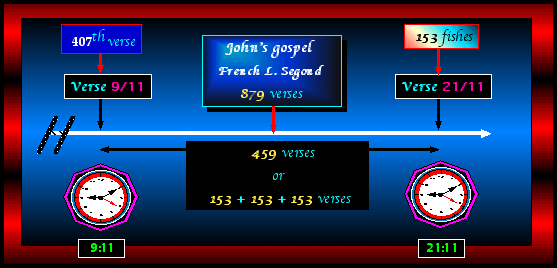It's high time to revisit another 21//11...

The French Louis Segond, Darby or US KJV versions (as a few examples) give a total of 153 + 153 + 153 verses if verses 9/11 and 21/11 are included.

" [...] Simon Peter went up, and drew the net to land full of great fishes, an hundred and fifty and three: and for all there were so many, yet was not the net broken. (John 21/11).

extracted from a 21/9-11 reference...

9 As soon then as they were come to land, they saw a fire of coals there, and fish laid thereon, and bread.
10 Jesus saith unto them, Bring of the fish which ye have now caught.
11 Simon Peter went up, and drew the net to land full of great fishes, an hundred and fifty and three: and for all there were so many, yet was not the net broken. (John 21/9-11).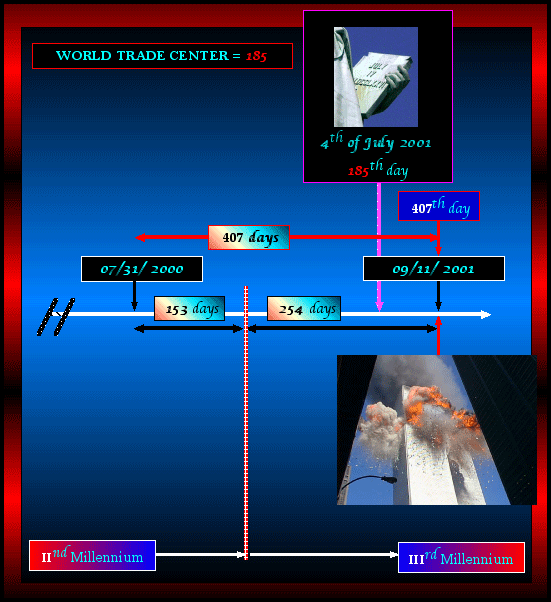153 days before the end of the IInd Millennium added to the (9:11 WTC events) 254th day give a total amount of 407 days.

"WORLD TRADE CENTER" = 72 + 48 + 65 = 185

The 4th of July 2001 was the 185th day of the 3rd Millennium...

254 = (2)3 + (5)3 + (4)3 = 8 + 125 + 64 = 197

197 = (1)3 + (9)3 + (7)3 = 1 + 729 + 343 = 1073

1073 = (1)3 + (0)3 + (7)3 + (3)3 = 1 + 0 + 343 + 27 = 371

371 = (3)3 + (7)3 + (1)3 = 27 + 343 + 1 = 371PAINS in SPAIN

911 days stand between "NYC WTC 9/11/2001 terrorism acts" and "MADRID 11/3/2001 terrorism acts".

six = 52 and six + six + six = 156 and 911 is the 156th prime number.

The Berlin Wall collapsed a 11th of September 1989 (11.9; written the French way). The 10th of March is the 69th day of a normal year and the 70th day of an overleap year. The 10th of March, 2004 (or day -297, 297= 144 (number of angel and man, measure of the Celestial Jerusalem) + 153 (other number attributed to Jesus Christ).
144... months later, 9/11 would occur!!!

This date between the 69th and the 70th day reminds us what the Bible says about the "Age of Grace" or "Age of Church" which stands just in-between the 69th week and the 70th week of DANIEL PROPHECY!

In the Louis Segond version of the Bible, dated 1917, 111 occurrences of the word "distress, emergency" (detresse in French) occur within 110 verses, which remind us of the 110 stories of the Twin Towers that collapsed... -111 days until the end of the year 2001, and let's don't forget the "emergency number" 911...

8 occurrences more of the word "emergencies" (plural form) occur in the Bible, making a total of... 119 occurrences (9/11, 11th of September), all occurring within 118 verses.

The reign of the Beast will last 1260 days, with the obligation to carry a mark which will also be a number, the number of a man who will rule the entire mankind. He will rule with his new kingdom, the NWO (in French "Nouvel Ordre Mondial", initials "N.O.M." (signifying "name" in French)) since the new ruler will also have a "name" ("NOM" in French)...

Our alphabet will become also ALPHA-B-E-T or ALPHA 2-5-20 (8 being the 2nd letter of the alphabet, E being the 5th letter, and T being the 20th letter of the alphabet)
There is a TV channel (US) named BET" with the pentagonal star. No comment! http://www.bet.com
"com" stands for "commercial".

"BET" and "AS" give "BEAST" in English ("BETE" in French). "AS" is French for "ACE".

In this language, this word "AS" has close ties with the "ACE" from the decks of cards. "AS" constitute also the initials of Arnold Schwarzenegger and "AS" can also be found on the Great Seal when using the existing pyramid to draw another one, thus creating a Star of David whose tips englobe, among the letters that form the word "MASON", A and S...

Jesus Christ alphanumerical value is 151. 151 is a prime number ("nombre premier" in French, alphanumerical value of "nombre premier" being.. 151) Holy Spirit is also 151

Area or ZONE 51 is Z -ONE-51. Z -ONE-51 or Z-1-51
Revelation.. 9/11 mentions two names of the Beast, ABADDON/APOLLYON = 151!
The Antichrist will be number ONE for 2520 days exactly. Notice that the UNO has become "UN" which means ONE in French. "Mais oui!"(yes, yes...)
PENTAGONE, PEN-TAG-ONE !
Pen: a pen to write with
Tag: a label,  a MARK
One: a person (in this case someone to be TAGGED)
Pentagone could also mean "The one-who-tags-people"(the Pen-tag one)

More and more, our walls are being "tagged". Walls are "MURS" in French.

MURS; The "M" and "R" of the French noun ""MuR"" are respectively the 13th and the 18th letter of the alphabet, which sends us right to... Revelation 13/18, where the number 666 is mentioned.

Another wall is making the headlines, the Security wall in Israel (+ "Weeping wall of Jerusalem), a very controversial construction built as a protection against terrorism. This same wall is being covered with Palestinian graffiti, many of which represent the Star of David, also named the "Star of the god Remphan", whose cult is being condemned in the New Testament, a highly occult sign, dating back to the 15th century, and associated with the Nazi Swastika. ( Yea, ye took up the tabernacle of Moloch, and the star of your god Remphan, figures which ye made to worship them: and I will carry you away beyond Babylon. Ac 7/43)

The French guru Rael wore this sign around his neck for 18 years, Rael, also known as His Holyness, ("Sa Saintete" in French, which initials remind us of the much famed Nazi "SS"), as an accusation against the Jewish people who is supposed to stand behind all the evil of the world.
How blind can people get!
(Ephesians 13/18) speaks of "tearing down the "Wall of Separation"...
This surrounding wall announces somehow the first birth pangs, tied up with the eschatological meaning of the number 153, as the following Bible reference puts it: (1 Tim 5;3)
(Rev. 12:1-4) mentions 12 stars
The European flag, of Marian inspiration, with its 12 revealing yellow stars ("revealing" or in its more accurate French version "reveal-matrix"(revelatrice, revel-matrice), which "matrix" (another word for "mother" ("Mère" in French) reminds us of the blasphemous Roman dogma and epithet of the Virgin MaRy, MaRy, MeRe (mother) of God!

This title is back in the actuality with the film "The Passion of Christ", and is tied together with the... 12th chapter of Revelation.

"MuR" (Wall) has an alphanumerical value of 52
WALL (its English translation) has an alphan. value of 53

In the Bible, (Eze 42:20), a wall marks the separation between the sacred and the profane."Wall" is mentioned 4 times in Rev. vv.24:12-17, also its length, which is 144 cubits, "the measure of man and angel".
The 2 different dating ways, the French way (the 11/9 dating way) and the anglo-saxon way (the 9/11 dating way) both reveal a worldwide conspiration, meant to lead toward the final FOURTH REICH. This movment's most important date, it's so-to-say an occult "HOLY DATE" started with Hitler and his dram to destroy Christianism and was celebrated on the 9th of November, (9/11), in commemoration of the "Beer Hall Putsch" of 1923.153 days separate July from the end of each year. And if we consider "J" for "Juillet/July", "A" for "Août/August", "S" for Septembre/September, "O" for "Octobre/October" and "N" for "Novembre/November", this "JASON/JONAS" sign period is exactly 153 days long.

Tony Blair came to the presidency of the UE Council on the 1rst of July 2005 and the 153rd hour of this "JASON" period has been darkened with the London July 7th tube and bus bombings.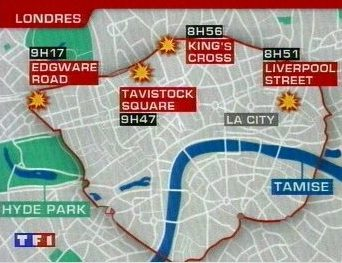7/7/05 TV TF1 Evening news screen capture

The bombings occurred between 8:51 and 9:47, 153rd and 154th French local time. One hour has to be cut off from the French hour to get the GMT local time. The summer time is two hours ahead the sun.

As a consequence, the bombing times become [153rd/154th] or [151st/153rd] solar time in France and [152nd/153rd] or [150th/151st] solar time in England.notes

Table of the first 100 multiplied numbers of 153:

 153 x 1 = 153 153 x 35 = 5355 153 x 69 = 10557 153 x 2 = 306 153 x 36 = 5508 153 x 70 = 10710 153 x 3 = 459 153 x 37 = 5661 153 x 71 = 10863 153 x 4 = 612 153 x 38 = 5814 153 x 72 = 11016 153 x 5 = 765 153 x 39 = 5967 153 x 73 = 11169 153 x 6 = 918 153 x 40 = 6120 153 x 74 = 11322 153 x 7 = 1071 153 x 41 = 6273 153 x 75 = 11475 153 x 8 = 1224 153 x 42 = 6426 153 x 76 = 11628 153 x 9 = 1377 153 x 43 = 6579 153 x 77 = 11781 153 x 10 = 1530 153 x 44 = 6732 153 x 78 = 11934 153 x 11 = 1683 153 x 45 = 6885 153 x 79 = 12087 153 x 12 = 1836 153 x 46 = 7038 153 x 80 = 12240 153 x 13 = 1989 153 x 47 = 7191 153 x 81 = 12393 153 x 14 = 2142 153 x 48 = 7344 153 x 82 = 12546 153 x 15 = 2295 153 x 49 = 7497 153 x 83 = 12699 153 x 16 = 2448 153 x 50 = 7650 153 x 84 = 12852 153 x 17 = 2601 153 x 51 = 7803 153 x 85 = 13005 153 x 18 = 2754 153 x 52 = 7956 153 x 86 = 13158 153 x 19 = 2907 153 x 53 = 8109 153 x 87 = 13311 153 x 20 = 3060 153 x 54 = 8262 153 x 88 = 13464 153 x 21 = 3213 153 x 55 = 8415 153 x 89 = 13617 153 x 22 = 3366 153 x 56 = 8568 153 x 90 = 13770 153 x 23 = 3519 153 x 57 = 8721 153 x 91 = 13923 153 x 24 = 3672 153 x 58 = 8874 153 x 92 = 14076 153 x 25 = 3825 153 x 59 = 9027 153 x 93 = 14229 153 x 26 = 3978 153 x 60 = 9180 153 x 94 = 14382 153 x 27 = 4131 153 x 61 = 9333 153 x 95 = 14535 153 x 28 = 4284 153 x 62 = 9486 153 x 96 = 14688 153 x 29 = 4437 153 x 63 = 9639 153 x 97 = 14841 153 x 30 = 4590 153 x 64 = 9792 153 x 98 = 14994 153 x 31 = 4743 153 x 65 = 9945 153 x 99 = 15147 153 x 32 = 4896 153 x 66 = 10098 153 x 100 = 15300 153 x 33 = 5049 153 x 67 = 10251 ... ... ... 153 x 34 = 5202 153 x 68 = 10404

153 blessings

given by Our Lord Jesus-Christ during His Life

 1. A leper ... (Matt 8/2) 1 2. A servant and a centurion... (Matt 8/5) 2 3. Peter's wife's mother ... (Matt 8/14) 1 4. Two possessed with devils... (Matt 8/18) 2 5. Four men bring to jesus a man sick of the palsy, lying on a bed. (Marc 2/3) (Matt 9/2) 5 6. Jairus and his daughter... (Matt 9/18) 2 7. A woman, which was diseased with an issue of blood twelve years ... (Matt 9/21) 1 8. Two blind men following Jesus ... (Matt 9/27) 2 9. A dumb man possessed with a devil ... (Matt 9/32) 1 10. The twelve apostles (- Judas Iscariot-)... (Matt 10/2) 11 11. The man which had his hand withered ... (Matt 12/10) 1 12. The man possessed with a devil, blind, and dumb ... (Matt 12/22) 1 13. James, and Joses, and Simon, and Judas, ... His brethren (Acts 1/14) (Matt 13/55) 4 14. The woman of Canaan with her daughter... (Matt 15/22) 2 15. The certain man and his son who is lunatick... (Matt 17/14) 2 16. Blind men departing from Jericho ... (Matt 20/30) 2 17. Simon the leper ... (Matt 26/6) 1 18. Mary (Lazarus' sister) ... (Matt 26/7) 1 19. The centurion ... (Matt 27/54) 1 20. Salome, the mother of Zebedee's children ... (Matt 27/56) 1 21. Mary, the mother of James and Joses... (Matt 27/56) 1 22. Mary Magdalene ... (Matt 27/56) 1 23. Joseph of Arimathaea... (Matt 27/57) 1 24. A man with an unclean spirit... (Mark 1/23) 1 25. The man that was deaf, and had an impediment in his speech... (Mark 7/32) 1 26. The blind man... (Mark 8/22) 1 27. A dead man carried out, the only son of his mother, a widow in Nain ... (Luke 7/12) 1 28. A woman in the city, which was a sinner... (Luke 7/37) 1 29. Joanna the wife of Chuza, and Susanna... (Luke 8/3) 2 30. One disciple -" Follow me." ... (Luke 9/59 1 31. The 70 disciples ... (Luke 10/1) 70 32. Martha ... (Luke 10/38) 1 33. The woman which had a spirit of infirmity eighteen years, ... (Luke 13/11) 1 34. A certain man which had the dropsy.. ... (Luke 14/2) 1 35. The 10 lepers ... (Luke 17/12) 10 36. The blind man nearing to Jericho... (Luke 18/35) 1 37. A very rich man named Zacchaeus, the chief among the publicans... (Luke 19/2) 1 38. And he touched his ear, and healed him... (Luke 22/51) 1 39. The malefactor on a cross... (Luke 23/43) 1 40. The two Emmaus disciples... (Luke 24/13) 2 41. Nicodemus... (John 3/1 1 42. A woman of Samaria... (John 4/4) 1 43. A certain nobleman, whose son was sick at Capernaum ... (John 4/46) 2 44. The impotent man in Bethesda... (John 5/1) 1 45. Woman taken in adultery, in the very act... (John 8/11) 1 46. The man which was blind from his birth... (John 9) 1 47. Lazarus ... (John 11) 1 48. Mary, Jesus' mother... (John 19/25) 1 Total : 153

" [...] But concerning the dead that they rise, have ye not read in the book of Moses, in the section of the bush, how God spoke to him, saying, I* am the God of Abraham, and the God of Isaac, and the God of Jacob? (Mr 12/26)

 L’an de grâce 1654, Lundi 23 novembre, ...//... depuis environ dix heures et demie du soir jusqu’à environ minuit et demie. FEU. Dieu d’Abraham, Dieu d’Isaac, Dieu de Jacob, non des philosophes et des savants. Certitude. Certitude. Sentiment, Joie, Paix. Dieu de Jésus-Christ, Deum meum et Deum vestrum.. Jean 20/17* " Ton Dieu sera mon Dieu " Oubli du monde et de tout, hormis Dieu. Il ne se trouve que par les voies enseignées dans l'Evangile. Grandeur de l'âme humaine. " Père juste, le monde ne t'a point connu, mais je t'ai connu ". Joie, Joie, Joie, pleurs de joie. Je m'en suis séparé : Dereliquerunt me fontem aquae vivae. " Mon Dieu me guérirez-vous? " Que je n'en sois pas séparé éternellement. " Cette vie est la vie éternelle, qu'ils te connaissent seul vrai Dieu, et celui que tu as envoyé, Jésus-Christ. " Jésus-Christ Jésus-Christ Je m'en suis séparé ; je l'ai fui, renoncé, crucifié, Jean 17** Que je n'en sois jamais séparé Il ne se conserve que par les voies enseignées dans l'Evangile. Renonciation totale et douce. Soumission totale et douce. Soumission totale à Jésus-Christ et à mon directeur. Eternellement en joie pour un jour d'exercice sur la terre. Non obliviscar sermones tuos. Amen. In the Year of Grace 1654, Monday, the 23rd of November ...//... From around 10.30 p.m. To around Midnight and half. FIRE God of Abraham, God of Isaac, God of Jacob Not of philosophers and scientists. Certitude. Certitude. Feeling. Joy. Peace. God of Jesus-Christ, Deum meum et Deum vestrum.. John 20/17* " Your God will be my God " Oblivion of the world and everything, save God. He is to be found in the ways that are taught in the Gospel. Greatness of human soul. " Father, the world did not know You, but I have Known You Joy, Joy, Joy, tears of Joy, I got away from Him : Dereliquerunt me fontem aquae vivae. " My God, will You ever cure me " Let me stand by Your side for ever This life is the Eternal life, Let them know You as the only True God, And the One You sent, Jesus-Christ " Jesus-Christ Jesus-Christ I got away from Him ; I fled away from Him, I abandoned Him, I crucified Him, John 17** Let me stand by His side for ever He is to be kept only through the ways that are taught in the Gospel. Total and sweet renunciation. Total and sweet renunciation. Total renunciation to Jesus-Christ and to my director Overjoyed forever for one day of exercise on earth. Non obliviscar sermones tuos. Amen. (Le " mémorial " de Blaise Pascal) (the " memorial " of Blaise Pascal)Bible and Numbers End Times Interpreted with the Help of the Bible, Numbers, and the French Language, by Anne E. Crews and Gérard Colombat (author of this website) "Was 9/11 supposed to happen, and is it related to the Rapture of the Christian Church? If so, how? Are all world events based on a coded numerical system, and if so, to whom does the coded numerical system point…the MESSIAH Jesus Christ? Bible and Numbers is a factual attempt to answer these lingering questions. Based on over thirty years of research by author Gérard Colombat, authors Anne E. Crews and Colombat proffer an intelligent and insightful theory in their quest for biblical truth. By juxtaposing a numerical system based on the modern calendar, sacred Bible numbers, and the phonetics and numerics of the French language, Colombat and Crews seek to define the occurrences of past events in conjunction with the present, offering that through the Bible and Numbers, questions of the future can be answered today. " 340 pages - \$18.99 (paperback) http://www.tatepublishing.com/bookstore/book.php?w=978-1-60462-558-5I read all the mails I get but it's quite impossible for me to reply. Thanks for your comprehension.Back to French Summary